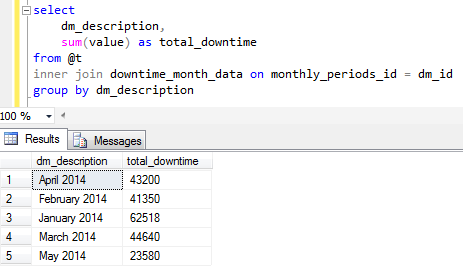﻿ SQL Period Calculation for Total Downtime per MonthSQL Server and T-SQL Development Tutorials Development resources, articles, tutorials, code samples, tools and downloads for ASP.Net, SQL Server, Reporting Services, T-SQL, Windows, AWS, SAP HANA and ABAP

# SQL Period Calculation for Total Downtime per Month

Period calculation in SQL or time calculations where developers sum specific events durations based on start and end times require coding by Transact-SQL developers. Generally SQL time duration calculation is difficult if you have to report time durations per pre-defined time blocks or time periods. And in those cases using DATEDIFF SQL function is not enough to get the result easily.

In this T-SQL tutorial, I'll try to prepare a simple report which lists server downtimes per each month. I had to use a reference table where time periods are stored. In this tutorial since downtimes per month is required to be listed, our reference time period table is going to include month data like start and end times, etc. You will see the details of the reference period database table and which fields are used how in the SQL script for the report.

First of all, create a helper table where we sql programmers frequently join to get data in their sql scripts. In this table we will store month information which forms the base of reporting in our SQL tutorial.

Now we can populate sample data in this base table. It is important that each month should be inserted into downtime_month_data base table in order. Otherwise, sql calculations will return wrong values.And now let's create sample data table for downtime calculation in our SQL tutorial scripts. This table can also be used for gap time calculation as well.

And enter sample sample data where downtime start and downtime end values are provided.I need two simple SQL user defined functions to use within SQL codes for calculating time periods. One of these two sql functions returns the period start time and the other one returns the period end time.

After all our database tables and user defined functions are created and sample tables are populated with example data, we can now create SQL script which will return downtimes grouped by the time periods expressed as months as follows.SQL Server